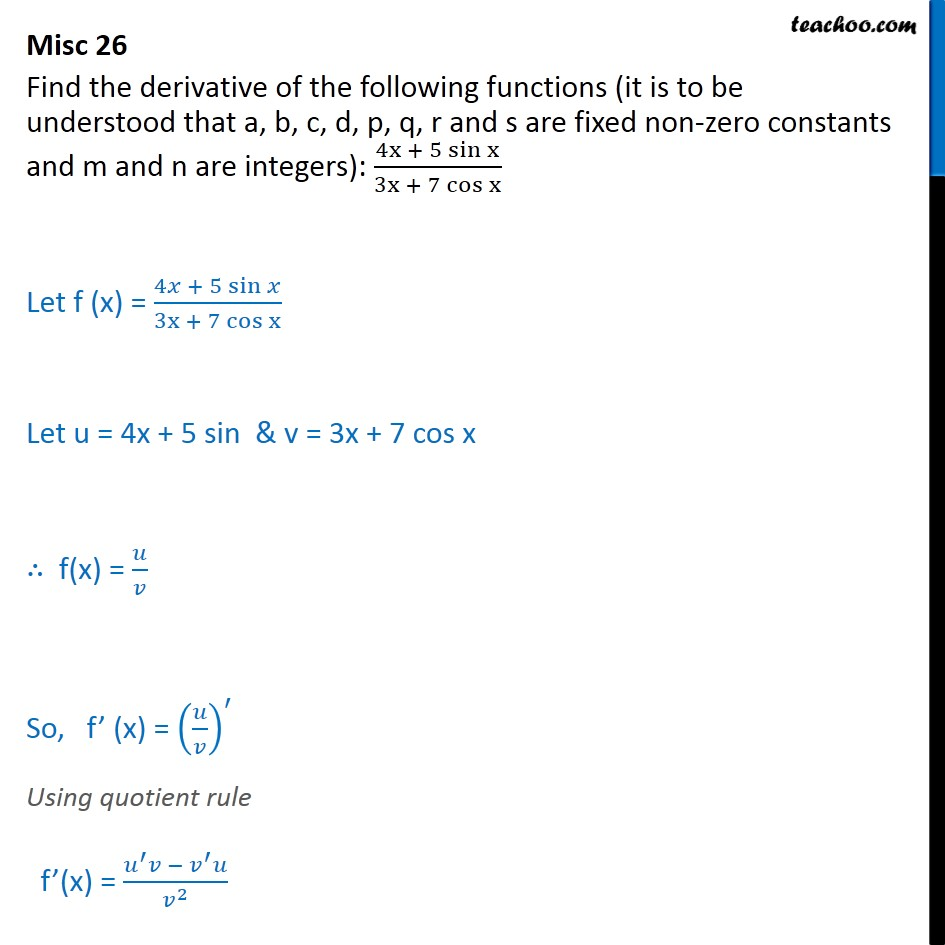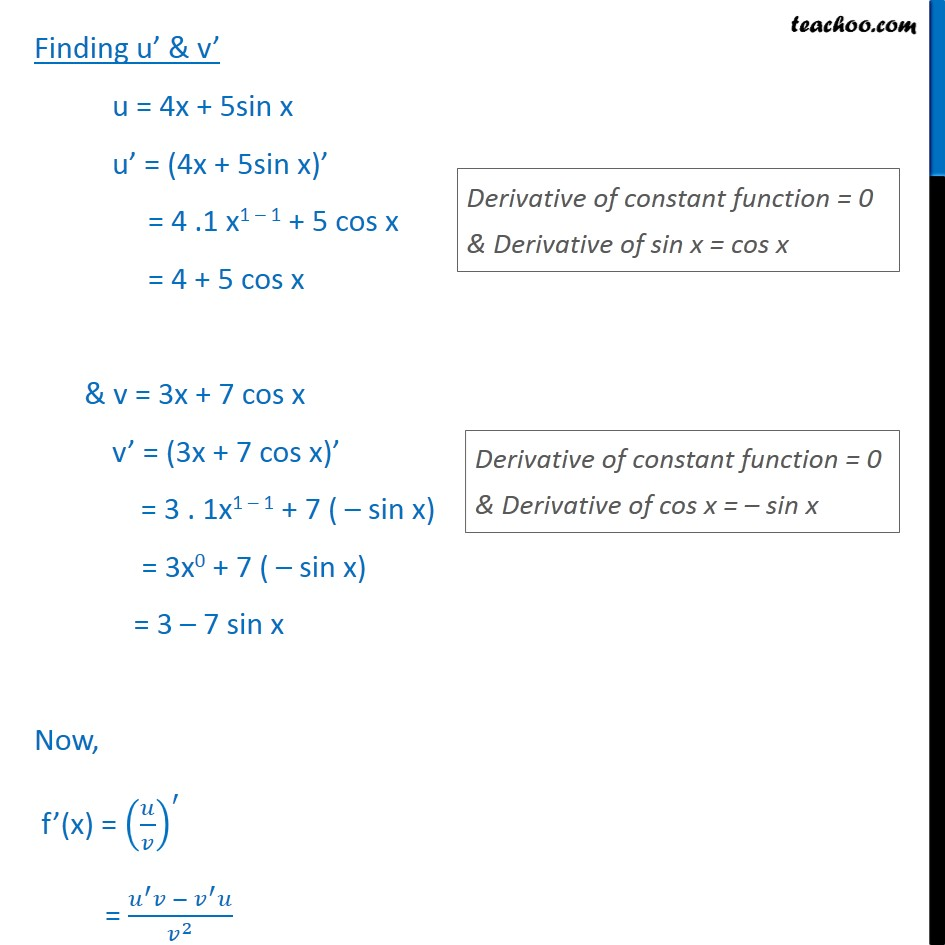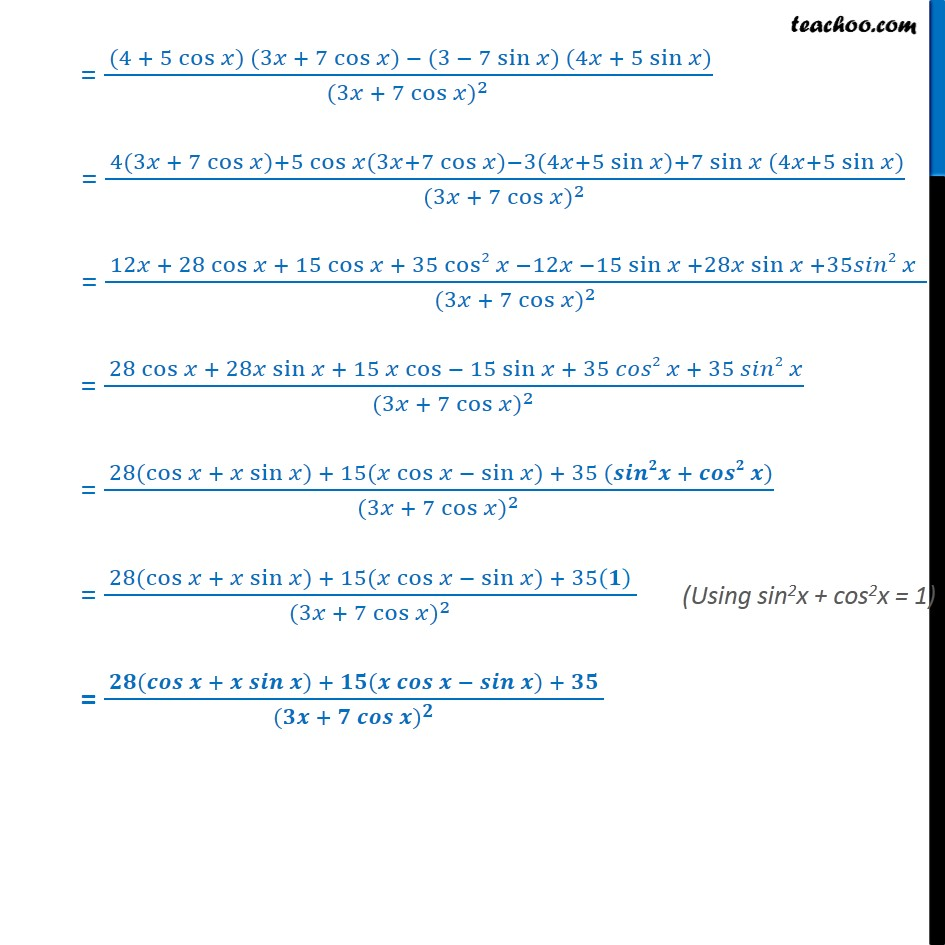Derivatives by formula - sin & cos

Chapter 12 Class 11 Limits and Derivatives
Concept wiseLearn in your speed, with individual attention - Teachoo Maths 1-on-1 Class

### Transcript

Misc 26 Find the derivative of the following functions (it is to be understood that a, b, c, d, p, q, r and s are fixed non-zero constants and m and n are integers): 4x + 5 sin﷮x﷯﷮3x + 7 cos﷮x﷯﷯ Let f (x) = 4𝑥 + 5 sin﷮𝑥﷯﷮3x + 7 cos x﷯ Let u = 4x + 5 sin & v = 3x + 7 cos x ∴ f(x) = 𝑢﷮𝑣﷯ So, f’ (x) = 𝑢﷮𝑣﷯﷯﷮′﷯ Using quotient rule f’(x) = 𝑢﷮′﷯𝑣 − 𝑣﷮′﷯𝑢﷮ 𝑣﷮2﷯﷯ Finding u’ & v’ u = 4x + 5sin x u’ = (4x + 5sin x)’ = 4 .1 x1 – 1 + 5 cos x = 4 + 5 cos x & v = 3x + 7 cos x v’ = (3x + 7 cos x)’ = 3 . 1x1 – 1 + 7 ( – sin x) = 3x0 + 7 ( – sin x) = 3 – 7 sin x Now, f’(x) = 𝑢﷮𝑣﷯﷯﷮′﷯ = 𝑢﷮′﷯𝑣 − 𝑣﷮′﷯𝑢﷮ 𝑣﷮2﷯﷯ = (4 + 5 cos﷮𝑥)﷯ (3𝑥 + 7 cos﷮𝑥)﷯ − (3 − 7 sin﷮𝑥)﷯ (4𝑥 + 5 sin﷮𝑥)﷯﷮ (3𝑥 + 7 cos﷮𝑥﷯)﷮2﷯﷯ = 4(3𝑥 + 7 cos﷮𝑥)+5 cos﷮𝑥(3𝑥+7 cos﷮𝑥)−3(4𝑥+5 sin﷮𝑥)+7 sin﷮𝑥 (4𝑥+5 sin﷮𝑥)﷯﷯﷯﷯﷯﷯﷮ (3𝑥 + 7 cos﷮𝑥﷯)﷮2﷯﷯ = 12𝑥 + 28 cos﷮𝑥 + 15 cos﷮𝑥 + 35 cos2﷮𝑥 −12𝑥 −15 sin﷮𝑥 ﷯+28𝑥 sin﷮𝑥 +35𝑠𝑖𝑛2 𝑥 ﷯ ﷯﷯﷯﷮ (3𝑥 + 7 cos﷮𝑥﷯)﷮2﷯﷯ = 28 cos﷮𝑥 + 28𝑥 sin﷮𝑥 + 15 𝑥 cos﷮− 15 sin﷮𝑥 + 35 𝑐𝑜𝑠2 𝑥 + 35 𝑠𝑖𝑛2 𝑥﷯﷯﷯﷯﷮ (3𝑥 + 7 cos﷮𝑥﷯)﷮2﷯﷯ = 28( cos﷮𝑥 + 𝑥 sin﷮𝑥) + 15(𝑥 cos﷮𝑥 − sin﷮𝑥) + 35 (𝒔𝒊𝒏𝟐𝒙 + 𝒄𝒐𝒔𝟐 𝒙)﷯﷯﷯﷯﷮ (3𝑥 + 7 cos﷮𝑥﷯)﷮2﷯﷯ = 28( cos﷮𝑥 + 𝑥 sin﷮𝑥) + 15(𝑥 cos﷮𝑥 − sin﷮𝑥) + 35 𝟏﷯ ﷯﷯﷯﷯﷮ (3𝑥 + 7 cos﷮𝑥﷯)﷮2﷯﷯ = 𝟐𝟖( 𝒄𝒐𝒔﷮𝒙 + 𝒙 𝒔𝒊𝒏﷮𝒙) + 𝟏𝟓(𝒙 𝒄𝒐𝒔﷮𝒙 − 𝒔𝒊𝒏﷮𝒙) + 𝟑𝟓 ﷯﷯﷯﷯﷮ (𝟑𝒙 + 𝟕 𝒄𝒐𝒔﷮𝒙﷯)﷮𝟐﷯﷯